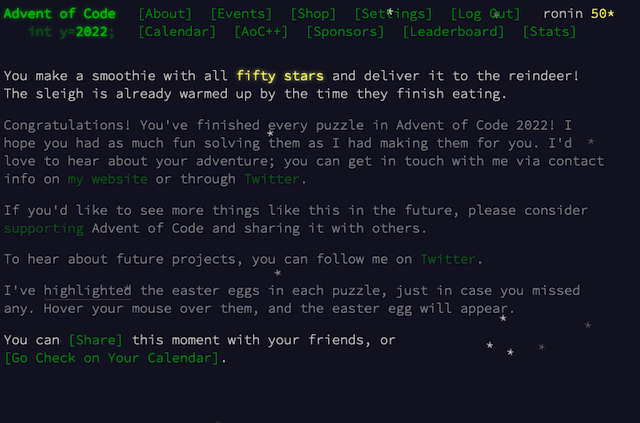# Advent of Code 2022, Day 25: Full of Hot Air

## Part A

At last Day 25. We need to implement conversion to and from decimal into some weird numeral system that has base 5, but values are -2, -1, 0, 1 and 2 instead of 0, 1, 2, 3 and 4. -2 is represented by = character and -1 is represented by - character.

In the puzzle this system is called SNAFU and here are some examples:

``````  Decimal          SNAFU
1              1
2              2
3             1=
4             1-
5             10
6             11
7             12
8             2=
9             2-
10             20
15            1=0
20            1-0
2022         1=11-2
12345        1-0---0
314159265  1121-1110-1=0
``````

I didn’t have any experienced with system system with negative values, but I recalled I saw one with -1, 0 and 1. I checked the list of numeral systems and found a system called balanced quinary, which is exactly what I was looking for. But there was nothing more except the name :) So I checked balanced ternary.

So the only thing necessary is to extend it for -2 and 2. To convert decimal to balanced ternary we need to do this:

• Convert number to plain ternary system (with base 3)
• Iterate over values from right to left, replace 2 with -1 and add one to the next value, replace 3 with 0 and add one to the next value

We need to extend it to base 5. The process is similar. We need to convert it to system with base 5. Then replace 3 with -2, 4 with -1 and 5 with 0. Finally replace -2 with = character and -1 with - character.

Here is my solution:

``````def decode_snafu(number)
value = 0
number.split("").reverse.each_with_index do |digit, power|
v = case digit
when "2"
2
when "1"
1
when "0"
0
when "-"
-1
when "="
-2
end

value += v * (5 ** power)
end

value
end

def encode_snafu(number)
values = []

while number > 0
values.push(number % 5)
number /= 5
end

index = 0
while index < values.size
if values[index] == 3
values[index] = -2

if values[index + 1].nil?
values.push(1)
else
values[index + 1] += 1
end
elsif values[index] == 4
values[index] = -1
if values[index + 1].nil?
values.push(1)
else
values[index + 1] += 1
end
elsif values[index] == 5
values[index] = 0
if values[index + 1].nil?
values.push(1)
else
values[index + 1] += 1
end
end

index += 1
end

values.map! do |digit|
if digit == -2
"="
elsif digit == -1
"-"
else
digit
end
end

values.reverse.join
end